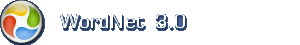Engelsk ordbok

Tips: Jokertegn må gjerne anvendes flere ganger i hvert søk.

Engelsk substantiv: law of nature

1. law of nature (om erkjendelse) a generalization that describes recurring facts or events in nature

Eksempler med tilsvarende betydningThe laws of thermodynamics.

Ord med samme betydning (synonymer)law

Mindre spesifikke uttrykkconcept, conception, construct

Mere spesifikke uttrykkall-or-none law, Archimedes' principle, Avogadro's hypothesis, Avogadro's law, Benford's law, Bernoulli's law, Bose-Einstein statistics, Boyle's law, Charles's law, Coulomb's Law, Dalton's law, Dalton's law, Dalton's law of partial pressures, distribution law, equilibrium law, exclusion principle, Fechner's law, Fermi-Dirac statistics, Gay-Lussac's law, Henry's law, Hooke's law, Hubble law, Hubble's law, Kepler's law, Kepler's law of planetary motion, Kirchhoff's laws, law of Archimedes, law of averages, law of chemical equilibrium, law of constant proportion, law of definite proportions, law of diminishing returns, law of effect, law of equivalent proportions, law of gravitation, law of large numbers, law of mass action, law of motion, law of multiple proportions, law of partial pressures, law of reciprocal proportions, law of thermodynamics, law of volumes, Mariotte's law, Mendel's law, Mendeleev's law, Newton's law, Newton's law of gravitation, Newton's law of motion, Ohm's law, Pascal's law, Pascal's law of fluid pressures, Pauli exclusion principle, periodic law, Planck's law, Planck's radiation law, power law, principle, principle of relativity, rule, Stevens' law, Stevens' power law, Weber's law, Weber-Fechner law

Omfatter disse overordnede uttrykkenetheory